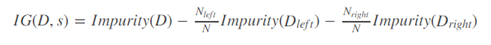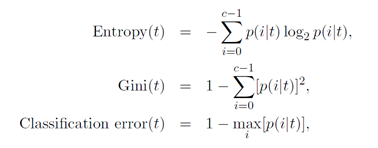# Global Data Science Forum

View Only

### Impurity Measures for Node Splitting

#### By Moloy De posted Thu July 30, 2020 08:34 PM

The decision tree is a greedy algorithm that performs a recursive binary partitioning of the feature space. The tree provides a class distribution in each leaf partition. Each partition is chosen greedily by selecting the best split from a set of possible splits, in order to maximize the information gain at a tree node. In other words, the split chosen at each tree node maximizes IG(D,s) where IG(D,s) is the information gain when a split s is applied to a dataset D.Following are the three popular Impurity Measures:Where p(i|t) is the probability or relative frequency of class i in node t.

Following is a comparison chart for above three measures in case of Binary Classification.QUESTION I: What are the three basic stop times for tree pruning?

QUESTION II: Why the decision tree is called a greedy algorithm?